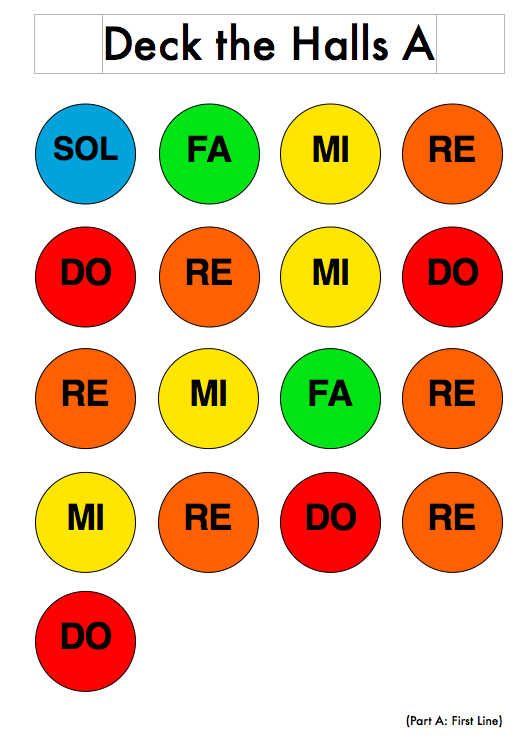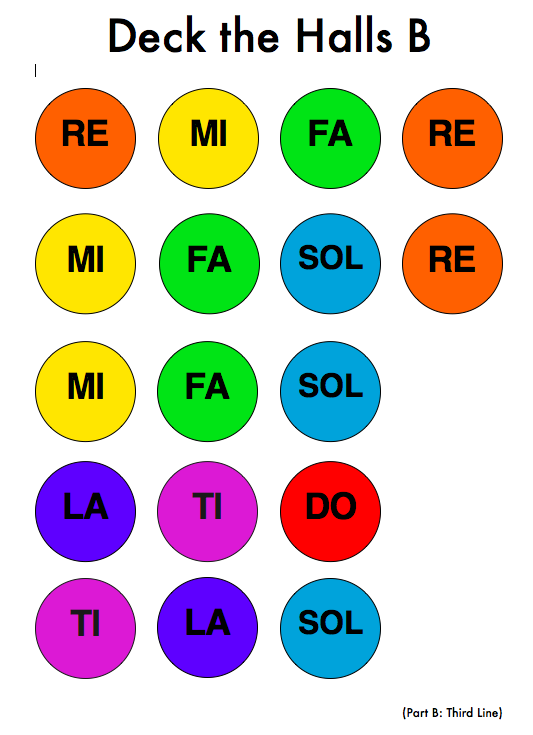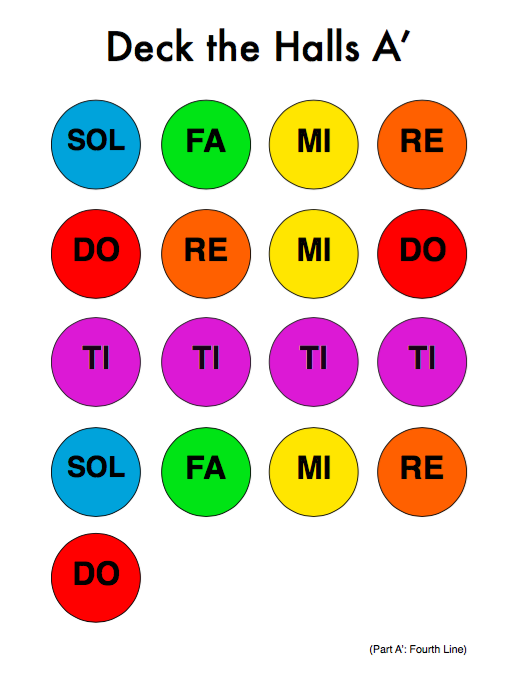# Deck the Halls

## s f m r d r m d r m f r m r d r d s f m r d r m d r m f r m r d r d r m f r m r s r m f s l t d’ t l s s f m r d r m d l l l l s f m r d

Click on the images below to go the a printable PDF.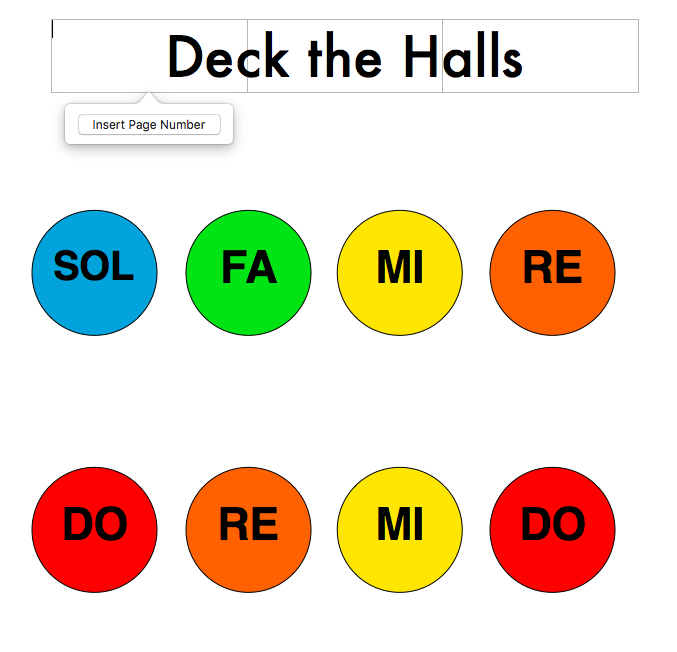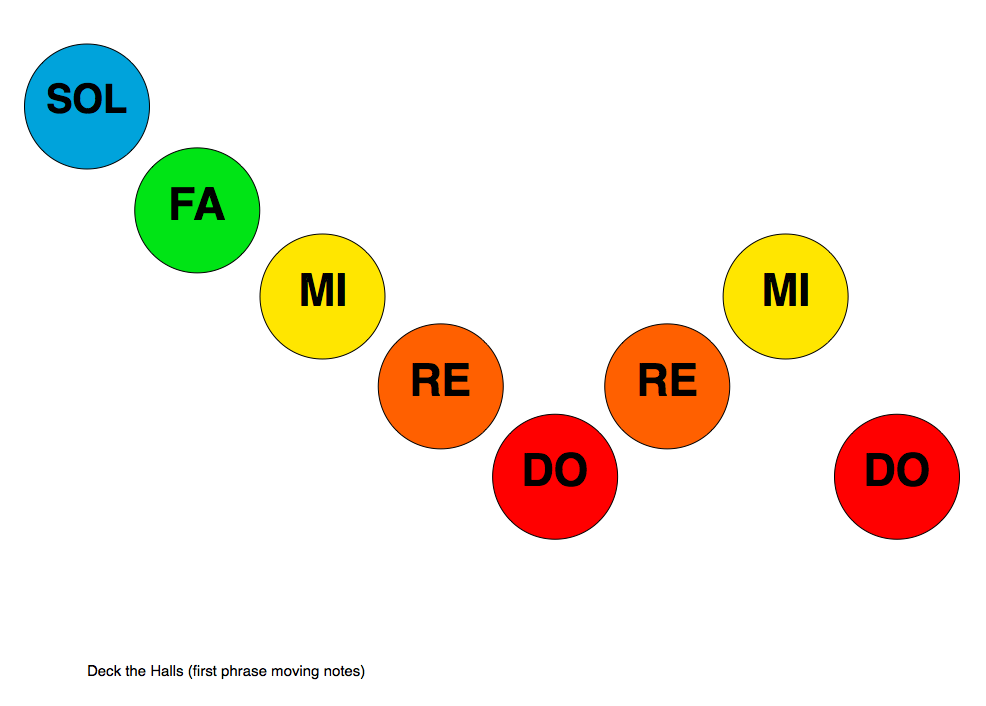Deck the Halls follows an AABA’ pattern (so you play the A part twice). The music is as follows for 1-octave resonator bells: